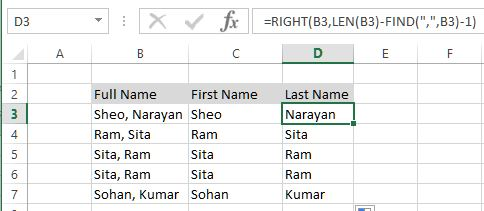Separate string in Excel

How to separate string from a specific characters in Excel?

In the previous post, we learnt about how to compare two strings (Case sensitive and Case insensitive) in Excel. In this post, we shall learn how to separate string from a specific character in Excel.

Let's take example of below data.

In B column we have Full Name of people separated by Comma (,). We need First Name and Last Name seprately into two different columns.

To get First Name, we have written '=LEFT(B3, (FIND(",",B3) - 1))' formula in C3 cell that

• finds the position of "," from B3 cell (using FIND function) and deduct 1 from that position
• takes the resultant number of characters from starting of the string using LEFT function.
The result is all characters from starting of the string till comma (,), this gives the First Name of the person.The same formula has been copied to other C column cells that gives above result.

To get Last Name, we have written '=RIGHT(B3,LEN(B3)-FIND(",",B3)-1)'  formula in D3 cell that

• finds the position of "," and deduct 1 and then deduct the resultant number from total length of the string
• takes the resultant number of characters from end of the string using RIGHT function.
The result is all characters from ending of the string till comma (,), this gives the Last Name of the person.The same formula has been copied to other D column cells that gives above result.

Views: 1887 | Post Order: 27